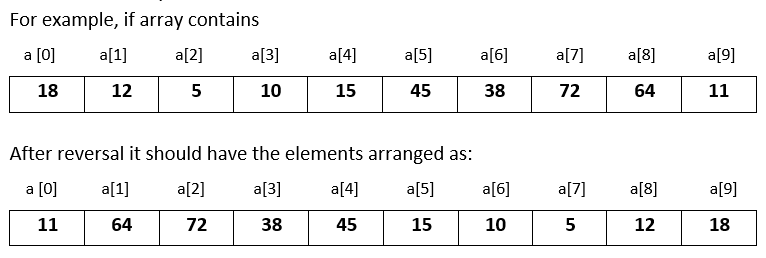# Input 10 numbers in integer array and reverse the original array in Python

###### Arrays - Question 7

In this question, we will see how to input 10 numbers in integer array and reverse the original array and print it on the screen in Python programming. To know more about arrays click on the arrays lesson.

Q7) Write a program in Python to input 10 numbers in integer array and reverse the original array and print it on the screen.#### Program

``````from array import *

t=0
a=array('i',[])

print('Enter 10 numbers')
for i in range(10):
a.append(int(input()))

for i in range(10//2):
t=a[i]
a[i]=a[9-i]
a[9-i]=t

print('\nModified array after reversal');
for n in a:
print(n,end=' ')``````

#### Output

```Enter 10 numbers
18
12
5
10
15
45
38
72
64
11

Modified array after reversal
11 64 72 38 45 15 10 5 12 18```